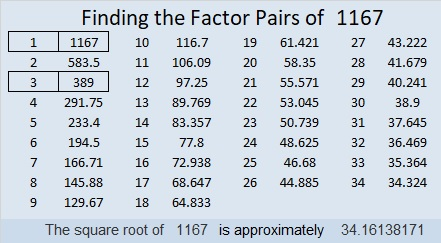# 1167 and Level 5

Will some of the tricky clues in this level 5 puzzle fool you? They won’t if you only write factors of which you are 100% sure. Always use logic. Never guess and check.Print the puzzles or type the solution in this excel file: 12 factors 1161-1173

Now I’ll write a little bit about the number 1167:

• 1167 is a composite number.
• Prime factorization: 1167 = 3 × 389
• The exponents in the prime factorization are 1 and 1. Adding one to each and multiplying we get (1 + 1)(1 + 1) = 2 × 2 = 4. Therefore 1167 has exactly 4 factors.
• Factors of 1167: 1, 3, 389, 1167
• Factor pairs: 1167 = 1 × 1167 or 3 × 389
• 1167 has no square factors that allow its square root to be simplified. √1167 ≈ 34.161381167 is the hypotenuse of a Pythagorean triple:
567-1020-1167 which is 3 times (189-340-389)

1167 is palindrome 5D5 in BASE 14 (D is 13 base 10)
because 5(14²) + 13(14) + 5(1) = 1167